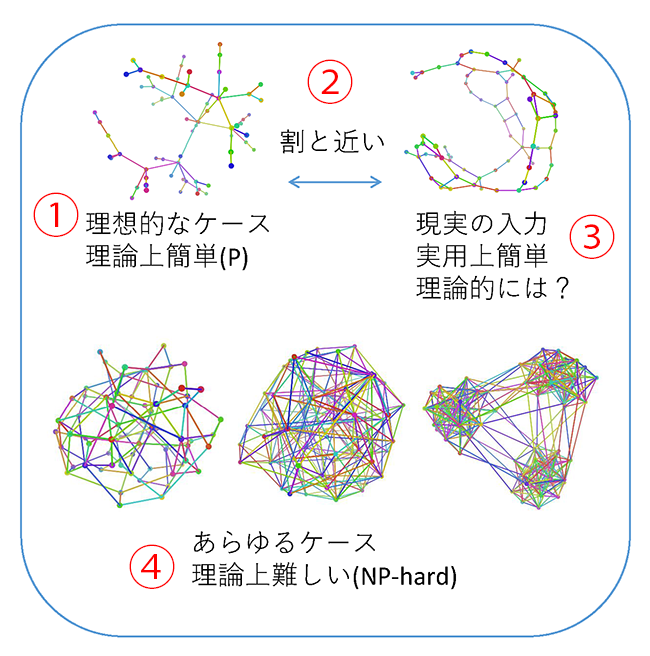# Research

## Principles of Informatics Research DivisionIWATA Yoichi
Principles of Informatics Research Division Assistant Professor
Doctoral Degrees: Doctor of Information Science and Technology (The University of Tokyo)
Research Fields: Mathematical Informatics
Detail: https://researchmap.jp/y_iwata/
WEB

### Introduction of research by science writer

#### Investigating the "difficulty" of problems that are hard to calculate

##### I want to better understand "difficulty"

My current research is focused on identifying the level of "difficulty" of NP-hard problems. NP-hard problems are problems that are difficult to handle in practice using computers because the computation time required increases so much when the scale of the problem increases. For example, "If you have a grid of n horizontal and n vertical roads, how many routes can you take from the top left to the bottom right without passing through the same point more than once?" If the grid consists of up to 4 rows/4 columns, this problem could be solved by hand by drawing a map and counting the number of routes. However, if there are 10 rows/10 columns, even a supercomputer would take several years to count the routes using this method. This is because the number of routes increases massively as the scale of the problem increases.
If a problem can be solved within a length of time proportional to the size of the problem, doubling the speed of the computer will mean that a problem of twice the size can be solved in the same amount of time. However, if a problem requires exponential time with respect to problem size, doubling the speed of the computer will only slightly increase the size of the problem that can be solved in the same amount of time. Therefore, improving algorithms is extremely important.

Although it is inevitable that the computation time of NP-hard problems will increase enormously when the scale of the problem increases, one of the two main topics that I am currently working on is developing calculation methods that reduce the amount of calculation, even if only slightly, and allow these problems to be solved precisely without settling for 'approximately.' The other main topic that I am working on is providing a theoretical explanation for methods that somehow operate at high speed for real input despite the amount of calculation being large in theory.

There are clearly limits to the calculation of NP-hard problems somewhere. However, we do not know where the limits are for many problems. Several years ago, a new calculation method for the famous NP-hard Hamiltonian Path problem was developed for the first time in fifty years, and this new method increased the speed of the calculation exponentially compared to the previous method. Incidentally, a faster method of calculating the problem that I gave as an example earlier has now been developed, and it currently takes a computer several tens of minutes to count the number of routes for a grid of 17 rows/17 columns.

##### Searching for the contact point between theory and reality1. Ideal case - Theoretically easy (P)
2. Relatively similar
3. Real input - Easy in practice. Theoretically easy?
4. All cases - Theoretically difficult (NP-hard)

In the real world, there exists a mixture of "problems that in theory are essentially unsolvable because the amount of calculation is extremely large," "problems that are realistically calculable, although the amount of calculation is slightly large," and "problems that require very little calculation." Reality is not perfect, even in terms of calculations. There are areas that are consistent with theory, areas that are partially consistent with theory, and areas that do not require theory to be introduced at all.

Computers are required to calculate problems that people truly desire to solve. Unlike the world of theory in which the possibilities of all inputs are considered, these kinds of problems are often given only inputs that have some sort of realistic structure. If what leads to "ease of solution" is identified, the structure of the background that determines "ease of solution" is analyzed, and a calculation method capable of using that structure is developed, after which it will be applicable to many similar problems.

Up until my doctoral course, I primarily carried out theoretical research, but from now on, I would also like to work on applying the findings that I have obtained through theoretical research to speed up calculations in the real world.